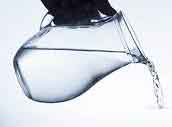Interesting Math Problem Math Problems Home  |  Home  |  Send Feedback

The Old Water Jugs Problem

Given two unmarked jugs, one which holds 7 liters,
and another which holds 11 liters,
an unlimited supply of water, and no need to conserve,
how do you measure exactly 6 liters?Problems of this type have been around for a long time,
but only recently did I realize that they are Diophantine equation
problems in disguise.

If we add 7's and subtract 11's, eventually we'll get to 6, or whatever other number is desired.

We just need to solve 7x - 11y = 6

I have another page which does that, but here I'll work this one out.

7 (x - y) - 4y = 6.
Let w = (x-y)
7w - 4y = 6
3w + 4 (w - y) = 6
By inspection, w = 2, y = 2 is a solution.
Then, x = w + y → x = 4.

If we fill the 7 liter jug 4 times,
and empty the 11 liter jug twice,
we'll have our 6 liters.

We proceed as follows:
ActionCount 7L jug11L jug
Start 0 0
Fill 7 (1) 7 0
Pour into 11 0 7
Fill 7 (2) 7 7
Pour into 11 3 11
Empty 11 [-1] 3 0
Pour into 11 0 3
Fill 7 (3) 7 3
Pour into 11 0 10
Fill 7 (4) 7 10
Pour into 11 6 11
At this point we have our 6, and don't need to carry out the last step, but it fits the equation better if we do.
Empty 11 [-2] 6 0

We can also solve this the other way around, using
11x - 7y = 6. In that case, our initial solution might be
x = -2, y = -4 (the negatives of the solution above)
but since you can't fill a jug "negative two times",
we can convert to positive values by adding 7 and 11, respectively,
to get
x = 5, y = 7,
which would mean fill the 11 five times, and empty the 7 seven times
Then, 5 * 11 - 7 * 7 = 55 - 49 = 6.
But that takes many more "fill, transfer, empty" steps than the above.

The general solution to 7x - 11y = 6 is
x = 4 + 11t,
y = 2 - 7t, for any integer t.
t x y
-3-29-19
-2-18-12
-1-7-5
042
1159
22616
The highlighted rows are the solutions mentioned above.